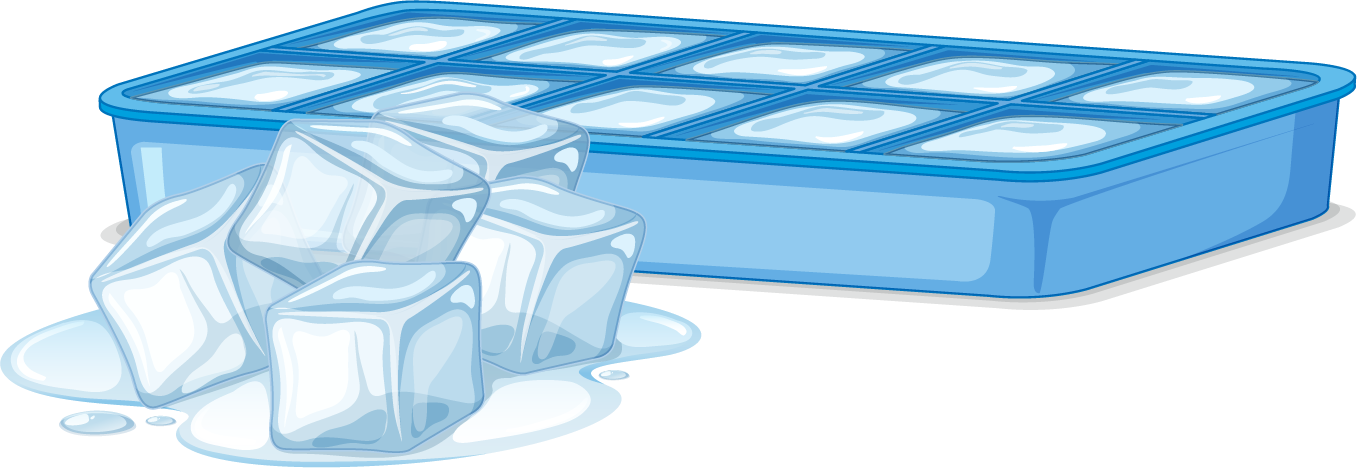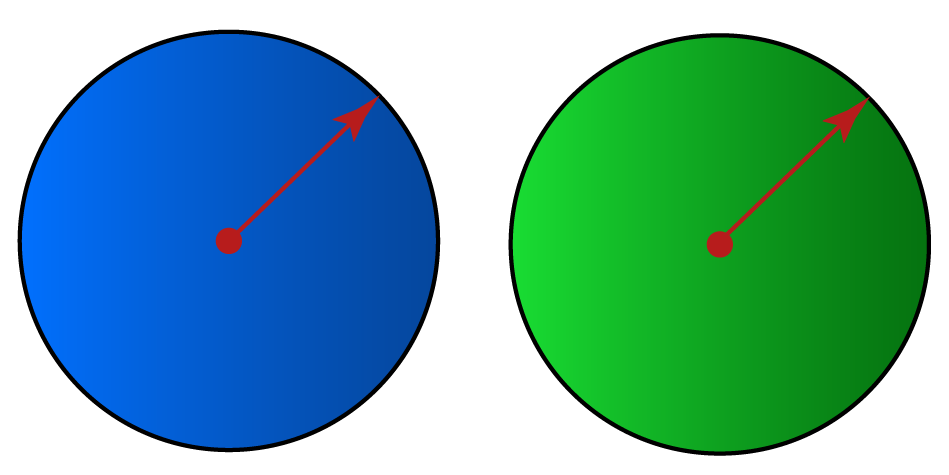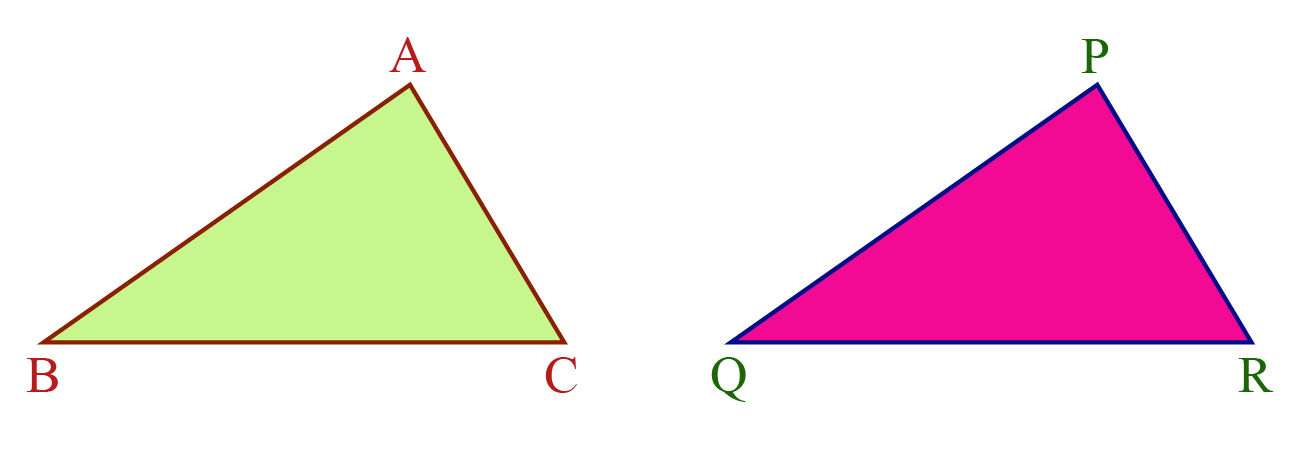# Congruent

Have you ever observed that two copies of a single photograph of the same size are identical?Similarly, ATM cards issued by the same bank are identical.Such figures are called congruent figures.

You may have noticed an ice tray in your refrigerator.

The molds inside the tray that is used for making ice are congruent.Have you struggled to replace a new refill in an older pen?

This could have happened because the new refill is not the same size as the one you want to replace.

Remember that whenever identical objects are to be produced, the concept of congruence is taken into consideration in making the cast.

In this chapter, we will learn about congruent shapes in the concept of congruent, using similar illustrative examples.

Check out the interactive simulation to explore more congruent shapes and do not forget to try your hand at solving a few interesting practice questions at the end of the page.

## What is Congruent?

### Congruent: Definition

The word "congruent" means equal in every aspect or figure in terms of shape and size.

Congruence is the term used to describe the relation of two figures that are congruent.

## What is Congruent Definition in Geometry?

Let us do a small activity.

Draw two circles of the same radius and place one on another.Do they cover each other completely?

Yes, they do.

Hence, we can say that they are congruent circles.

### Examples

Use the following simulation to explore more congruent shapes.

## How are Triangles Congruent?

Now let's discuss the congruence of two triangles.

Look at $$\Delta ABC$$ and $$\Delta PQR$$ below.Now, measure the sides and the angles of the triangles.

What do you observe?

Do they measure the same?

If you place $$\Delta ABC$$ on top of $$\Delta PQR$$, would they superimpose?

Yes!

We then say the two triangles are congruent.

We can represent this in a mathematical form using the congruent symbol.

$$\Delta ABC \cong \Delta PQR$$

This means $$A$$ falls on $$P$$, $$B$$ falls on $$Q$$ and $$C$$ falls on $$R$$.

Also, $$AB$$ falls on $$PQ$$, $$BC$$ falls on $$QR$$ and $$AC$$ falls on $$PR$$.

This indicates that the corresponding parts of congruent triangles are equal.

Congruent Parts of $$\mathbf{\Delta ABC}$$ and $$\mathbf{\Delta PQR}$$
Corresponding Vertices

A and P

B and Q

C and R

Corresponding Sides

$$\overline{AB}$$ and $$\overline{PQ}$$

$$\overline{BC}$$ and $$\overline{QR}$$

$$\overline{AC}$$ and $$\overline{PR}$$

Corresponding Angles

$$\angle A$$ and $$\angle P$$

$$\angle B$$ and $$\angle Q$$

$$\angle C$$ and $$\angle R$$

Remember that it is incorrect to write $$\Delta BAC \cong \Delta PQR$$ because $$A$$ corresponds to $$P$$, $$B$$ corresponds to $$Q$$ and $$C$$ corresponds to $$R$$.

## Let's Summarize

We hope you enjoyed learning about Congruent with the simulations and practice questions. Now you will be able to easily solve problems on congruent shapes.

## About Cuemath

At Cuemath, our team of math experts is dedicated to making learning fun for our favorite readers, the students!

Through an interactive and engaging learning-teaching-learning approach, the teachers explore all angles of a topic.

Be it worksheets, online classes, doubt sessions, or any other form of relation, it’s the logical thinking and smart learning approach that we, at Cuemath, believe in.

## 1. How do you prove a shape is congruent?

Two shapes are congruent if they are exact copies of each other and when superimposed, they cover each other exactly.

## 2. What is another word for congruent?

Congruent shapes are also called coinciding shapes.

## 3. What makes an angle congruent?

Two angles are congruent if their measures are exactly the same.

More Important Topics
Numbers
Algebra
Geometry
Measurement
Money
Data
Trigonometry
Calculus